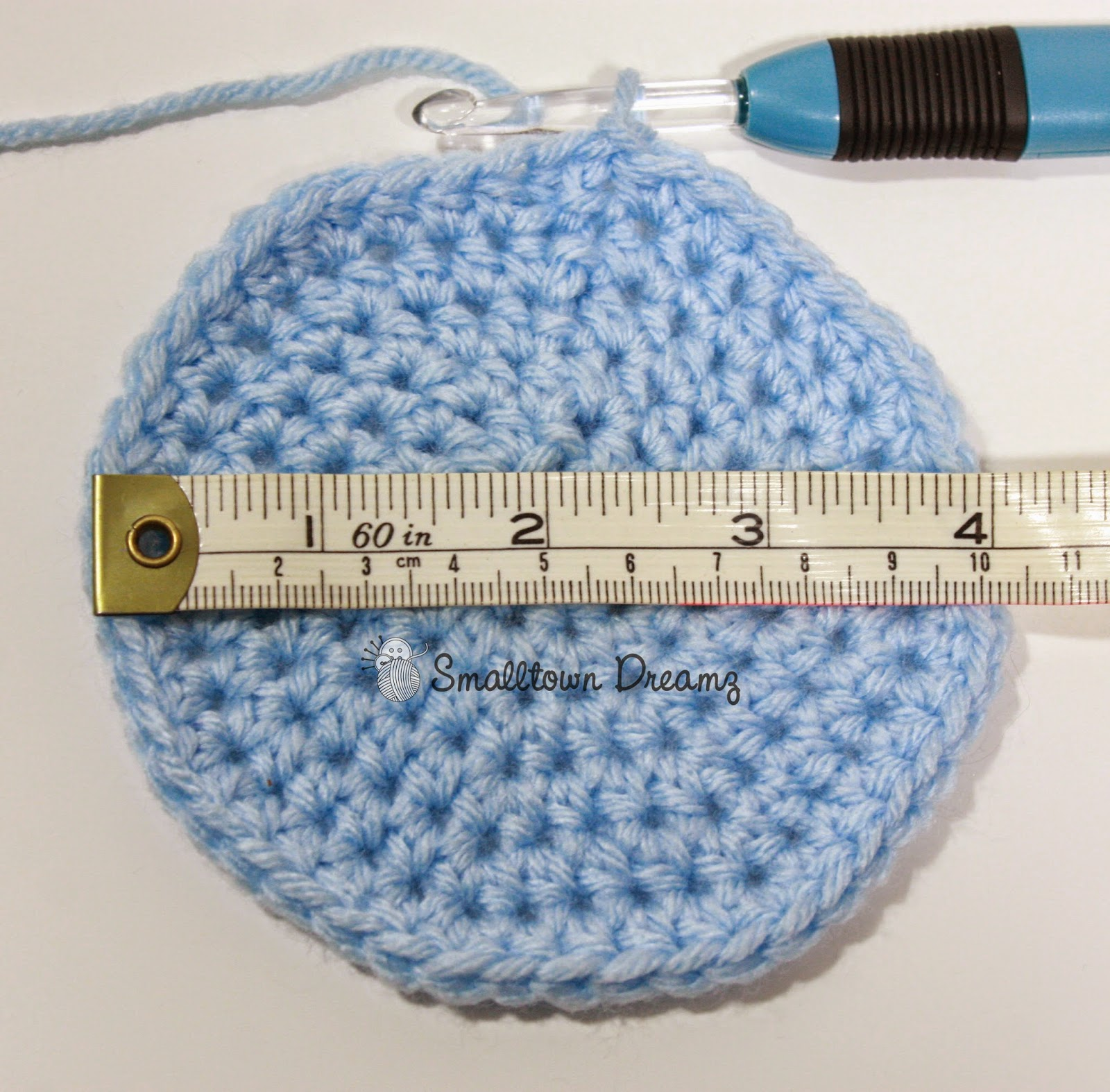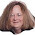## Monday, December 1, 2014

### Sizing Formula for Crochet Hats

Recently over the holidays I ran into a fellow crocheter who had some awesome knowledge to share. I for one LOVE math which is why I think crochet designing is so much fun for me. However I was INTRIGUED when she told me that there is a math formula to figure out hat sizes. Seeing how this is something that I am really interested in and was finding it difficult to determine sizes, I started taking notes.

After I got home I started crunching numbers and comparing the results with other sizing charts and I was floored that the formulas were pretty accurate. I will for sure be keeping this in mind for the rest of my crocheting life. HAHA.

So this is how she broke it down for me.Crochet Circle
Formulas for finding crochet hat sizes.

Circumference (divided by) 3.14 = Size of Crochet Circle

Circumference (divided by) 10 = ? (multiplied by) 4 = Hat Height

Circumference (divided by) 2 = Hat width

*** These formulas will work with Most hat patterns but not all depending on the pattern type***

For example......

0-3 month old - Circumference = 12 - 14 inches

Circle Size
12 / 3.14 = 3.8 (round nearest whole number or .5)
14/ 3.14 = 4.4 (round nearest whole number or .5)
so your circle size would be 4 inches to 4.5 inches

Hat Height
12 / 10 = 1.2 * 4 = 4.8 (round nearest whole number or .5)
14 / 10 = 1.4 * 4 = 5.6 (round nearest whole number or .5)
So your hat height would be 5 inches to 5.5 inches

Hat Width
12 / 2 = 6
14 / 2 = 7
So your hat width would be 6 inches to 7 inches

Neat huh?!?!?!?!

For those that don't enjoy math like I do I have it all broken down for you below. I hope you enjoy!!!!

Newborn - Circumference = 12 inches

Circle Size
12 / 3.14 = 3.8 (round to the nearest whole number, .75, .50 or .25)
so your circle size would be 4 inches to

Hat Height
12 / 10 = 1.2 * 4 = 4.8 (round nearest whole number, .75, .50 or .25)
So your hat height would be 5 inches

Hat Width
12 / 2 = 6
So your hat width would be 6 inches to

0-3 month old - Circumference = 12 - 14 inches

Circle Size
12 / 3.14 = 3.8 (round nearest whole number, .75, .50 or .25)
14/ 3.14 = 4.4 (round nearest whole number, .75, .50 or .25)
so your circle size would be 4 inches to 4.5 inches

Hat Height
12 / 10 = 1.2 * 4 = 4.8 (round nearest whole number, .75, .50 or .25)
14 / 10 = 1.4 * 4 = 5.6 (round nearest whole number, .75, .50 or .25)
So your hat height would be 5 inches to 5.5 inches

Hat Width
12 / 2 = 6
14 / 2 = 7
So your hat width would be 6 inches to 7 inches

3-6 month old - Circumference = 14 - 16 inches

Circle Size
14/ 3.14 = 4.4 (round nearest whole number, .75, .50 or .25)
16/ 3.14 = 5.0 (round nearest whole number, .75, .50 or .25)
so your circle size would be 4.5 inches to 5 inches

Hat Height
14 / 10 = 1.4 * 4 = 5.6 (round nearest whole number, .75, .50 or .25)
16 / 10 = 1.6 * 4 = 6.4 (round nearest whole number, .75, .50 or .25)
So your hat height would be 5.5 inches to 6.5 inches

Hat Width
14 / 2 = 7
16 / 2 = 8
So your hat width would be 7 inches to  8 inches

6-12 month old - Circumference =  16 - 18 inches

Circle Size
16/ 3.14 = 5.0 (round nearest whole number, .75, .50 or .25)
18/ 3.14 = 5.7 (round nearest whole number, .75, .50 or .25)
so your circle size would be 5 inches to 6 inches

Hat Height
16 / 10 = 1.6 * 4 = 6.4 (round nearest whole number, .75, .50 or .25)
18 / 10 = 1.8 * 4 = 7.2 (round nearest whole number, .75, .50 or .25)
So your hat height would be 6.5 inches to 7.25 inches

Hat Width
16 / 2 = 8
18 / 2 = 9
So your hat width would be 8 inches to 9 inches

1-3 year old - Circumference = 18 - 20 inches

Circle Size
18/ 3.14 = 5.7 (round nearest whole number, .75, .50 or .25)
20/ 3.14 = 6.36 (round nearest whole number, .75, .50 or .25)
so your circle size would be   6 inches to 6.5 inches

Hat Height
18 / 10 = 1.8 * 4 = 7.2 (round nearest whole number, .75, .50 or .25)
20 / 10 = 2.0 * 4 = 8.0 (round nearest whole number, .75, .50 or .25)
So your hat height would be 7.25 inches to 8 inches

Hat Width
18 / 2 = 9
20 / 2 = 10
So your hat width would be 9 inches  to 10 inches

3-10 year old - Circumference =  20 - 22 inches

Circle Size
20 / 3.14 = 6.3 (round nearest whole number, .75, .50 or .25)
22 / 3.14 = 7.0 (round nearest whole number, .75, .50 or .25)
so your circle size would be 6.5 inches to 7 inches

Hat Height
20 / 10 = 2.0 * 4 = 8.0 (round nearest whole number, .75, .50 or .25)
22 / 10 = 2.2 * 4 = 8.8 (round nearest whole number, .75, .50 or .25)
So your hat height would be 8 inches  to 9 inches

Hat Width
20 / 2 = 10
22 / 2 = 11
So your hat width would be 10 inches to 11 inches

Teen to Adult - Circumference =   22 - 24  inches

Circle Size
22 / 3.14 = 7.0 (round nearest whole number, .75, .50 or .25)
24 / 3.14 = 7.6 (round nearest whole number, .75, .50 or .25)
so your circle size would be 7 inches  to  7.75 inches

Hat Height
22 / 10 = 1.8 * 4 = 8.8 (round nearest whole number, .75, .50 or .25)
24 / 10 = 2.4 * 4 = 9.6 (round nearest whole number, .75, .50 or .25)
So your hat height would be 9 inches to 9.5 inches

Hat Width
22 / 2 = 11
24 / 2 = 12
So your hat width would be 11 inches to 12 inches

I hope that all makes sense and I hope this helps with sizing your hats in the future!!!

In the mean time Here is a hat I made for my oldest son recently to match his new winter jacket. The pattern is the Griffin Hat by Sincerely Pam

I look forward to finding more tips and tricks to share with you this next year along with a few other fun things. Until then...................

Happy Crafting

#### 1 comment:

1.When I first started making hats I remembered there was a formula to find the circumference of a circle when you have the diameter. I thought I remembered it, but checked with a math teacher friend.

It makes the sizing process so easy when you follow: Circumference = diameter x 3.14 . . . (or pi to have fin with people.)

You are right, some times it can be a little off, so I re=measure my hats when I am 2 rnds into the body and adn if needed.

GREAT POST ! ! ! ! !

Thank you for taking the time to comment

### Looking Forward.....

As I sit here and dust off the cobwebs to my tiny ghost town of a blog, I am excited to make a fresh start and slowly get back to what...